A popular and useful .csv file containing information on loan applicants at German banks is available from many sites on the web. The file contains 20 pieces of information on 1000 applicants.

The following code can be used to determine if an applicant is credit worthy and if he (or she) represents a good credit risk to the lender. Several methods are applied to the data to help make this determination. We will look at them in this case.

Please note, the data file used in this example exists in different forms around the web, so it might be necessary to perform some data manipulation to prepare it for the analysis.

url <- 'https://onlinecourses.science.psu.edu/stat857/sites/onlinecourses.science.psu.edu.stat857/files/german_credit.csv'
credit <- read.csv(url, header = TRUE, sep = ',')

This bit of code performs a small manipulation on the data to prepare it for the analysis. Otherwise, an error results because of the four levels of factors found in one of the columns in some files. There weren’t many in the file I worked from, just a handful of 1000 rows, and the results are not impacted materially.

Basically, any level 4 response is coverted to level 3. What matters is the code made R happy and the analysis went ahead.

credit$No.of.Credits.at.this.Bank[credit$No.of.Credits.at.this.Bank == 4] <- 3

Take a quick look at the data to get a sense of what we are working with.

str(credit)
## 'data.frame':    1000 obs. of  21 variables:
##  $Creditability : int 1 1 1 1 1 1 1 1 1 1 ... ##$ Account.Balance                  : int  1 1 2 1 1 1 1 1 4 2 ...
##  $Duration.of.Credit..month. : int 18 9 12 12 12 10 8 6 18 24 ... ##$ Payment.Status.of.Previous.Credit: int  4 4 2 4 4 4 4 4 4 2 ...
##  $Purpose : int 2 0 9 0 0 0 0 0 3 3 ... ##$ Credit.Amount                    : int  1049 2799 841 2122 2171 2241 3398 1361 1098 3758 ...
##  $Value.Savings.Stocks : int 1 1 2 1 1 1 1 1 1 3 ... ##$ Length.of.current.employment     : int  2 3 4 3 3 2 4 2 1 1 ...
##  $Instalment.per.cent : int 4 2 2 3 4 1 1 2 4 1 ... ##$ Sex...Marital.Status             : int  2 3 2 3 3 3 3 3 2 2 ...
##  $Guarantors : int 1 1 1 1 1 1 1 1 1 1 ... ##$ Duration.in.Current.address      : int  4 2 4 2 4 3 4 4 4 4 ...
##  $Most.valuable.available.asset : int 2 1 1 1 2 1 1 1 3 4 ... ##$ Age..years.                      : int  21 36 23 39 38 48 39 40 65 23 ...
##  $Concurrent.Credits : int 3 3 3 3 1 3 3 3 3 3 ... ##$ Type.of.apartment                : int  1 1 1 1 2 1 2 2 2 1 ...
##  $No.of.Credits.at.this.Bank : num 1 2 1 2 2 2 2 1 2 1 ... ##$ Occupation                       : int  3 3 2 2 2 2 2 2 1 1 ...
##  $No.of.dependents : int 1 2 1 2 1 2 1 2 1 1 ... ##$ Telephone                        : int  1 1 1 1 1 1 1 1 1 1 ...
##  $Foreign.Worker : int 1 1 1 2 2 2 2 2 1 1 ... You probably notice immediately three columns stand out. We are going to eliminate them: “Duration of Credit (months)”, “Credit Amount”, and “Age”. Why? We are trying to focus in this exercise on classifications or categories of data that serve as indicators of credit worthiness. These are scores rather than hard numbers. Does the applicant have a phone? Is the applicant married? Is there a co-signer? How long has the applicant lived at the same address? These sorts of things. What’s important about these factors is we know how they relate to lending decisions. Good credit is associated with certain combinations of factors to the point we use probabilities to classify new applicants by their characteristics. In the data, the answers to these questions are not “Yes” or “No” or “Ten Years”. The answers are grouped into broader classifications. Take a look at the data and column headers and you will see what I mean. What we need to do then is remove the truly numeric data (duration, amount, and age) and preserve the categorical factors. We do that by creating an object that excludes selected columns. S <- c(1, 2, 4, 5, 7, 8, 9, 10, 11, 12, 13, 15, 16, 17, 18, 19, 20) Then we create a short function to convert integers to factors. for(i in S) credit[, i] <- as.factor(credit[, i]) Now that we have the data in useful shape, we can begin to apply different analytical methods. #### Method One: Logistic Regression The first step is to create our practice data set and our test data set. THe practice set is used for training the model. The test set is used to evaluate model accuracy. We are going to create a number of models so it is necessary to give them numerical designations (1, 2, 3, etc.). We can break the data sets into any sizes we like, even 50-50, but here we use a one-third, two-thirds split. i_test1 <- sample(1:nrow(credit), size = 333) i_calibration1 <- (1:nrow(credit))[-i_test1] At this stage, we will perform a logistic regression using the glm() function. We start with the practice set, i_calibration1. Here, we will be selective with the variables we use in the model. We’ll change this in a bit but for now just use five to determine the value of Creditability. set.seed(1) LogisticModel.1 <- glm(Creditability ~ Account.Balance + Payment.Status.of.Previous.Credit + Purpose + Length.of.current.employment + Sex...Marital.Status, family = binomial, data = credit[i_calibration1, ]) That done, we can move on to fitting the model we just created to the test set, i_test1, and prepare to make our first prediction. fitLog1 <- predict(LogisticModel.1, type = 'response', newdata = credit[i_test1, ]) We have fitted our model. Now we will use the ROCR package to create predictions and measure performance in terms of Area Under the Curve (AUC). The greater the AUC measure, the better our model is performing. library(ROCR) pred1 <- prediction(fitLog1, credit$Creditability[i_test1])
perf1 <- performance(pred1, 'tpr', 'fpr')

Let’s plot our results.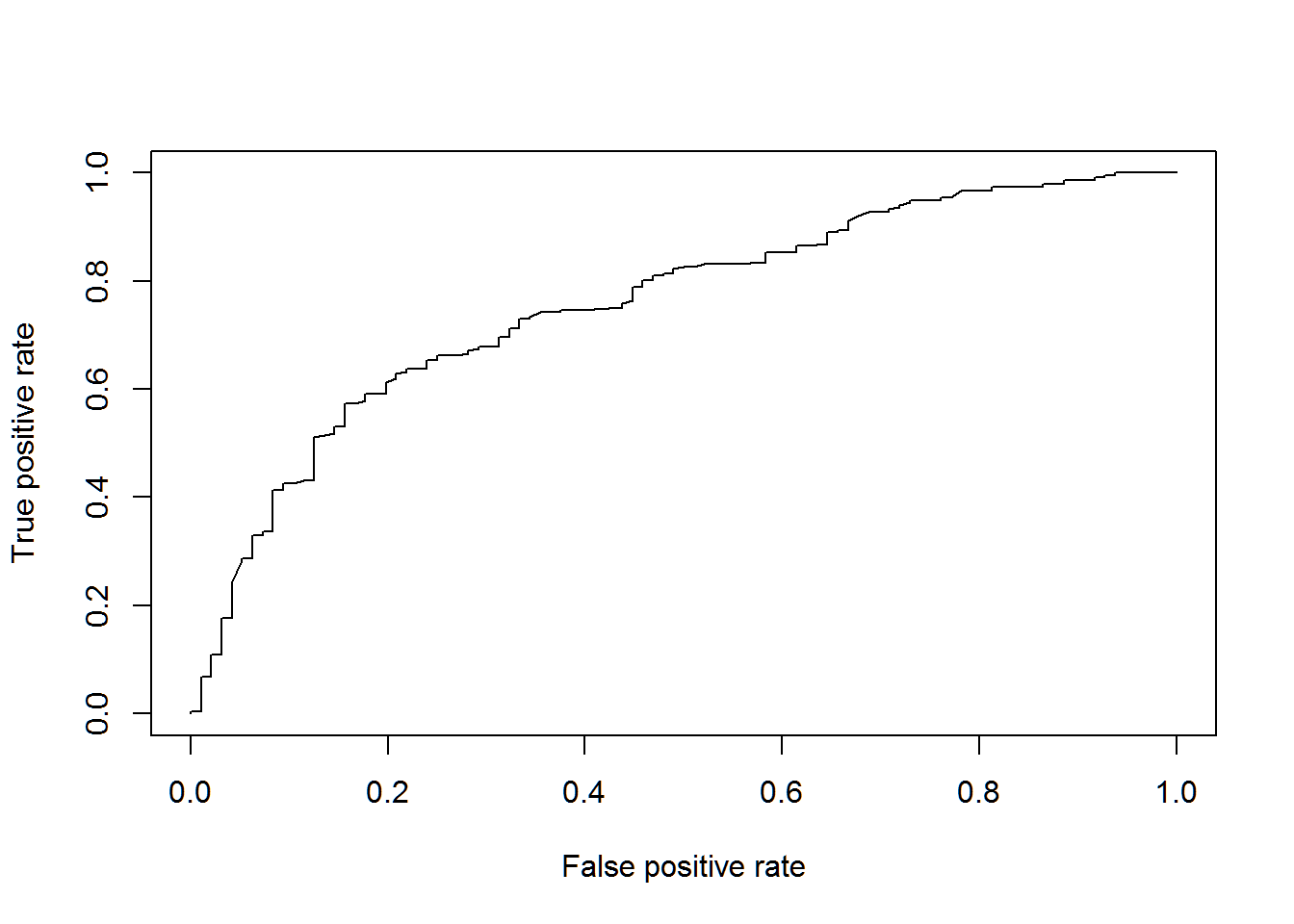And we’ll wrap this part up by finding the AUC.

AUCLog1 <- performance(pred1, measure = 'auc')@y.values[]
AUCLog1
##  0.7564829

That’s not a bad result, but let’s see if we can do better with a different method.

#### Method Two: An Alternative Logistic Model

In this approach, we will build a second Logistic Model to make use of all the variables in our data set. The steps are the same as in the first model above so we can dispense with much of the commentary.

set.seed(1)
LogisticModel.2 <- glm(Creditability ~ ., family = binomial, data = credit[i_calibration1, ])
fitLog2 <- predict(LogisticModel.2, type = 'response', newdata = credit[i_test1, ])
pred2 <- prediction(fitLog2, credit$Creditability[i_test1]) perf2 <- performance(pred2, 'tpr', 'fpr') plot(perf2)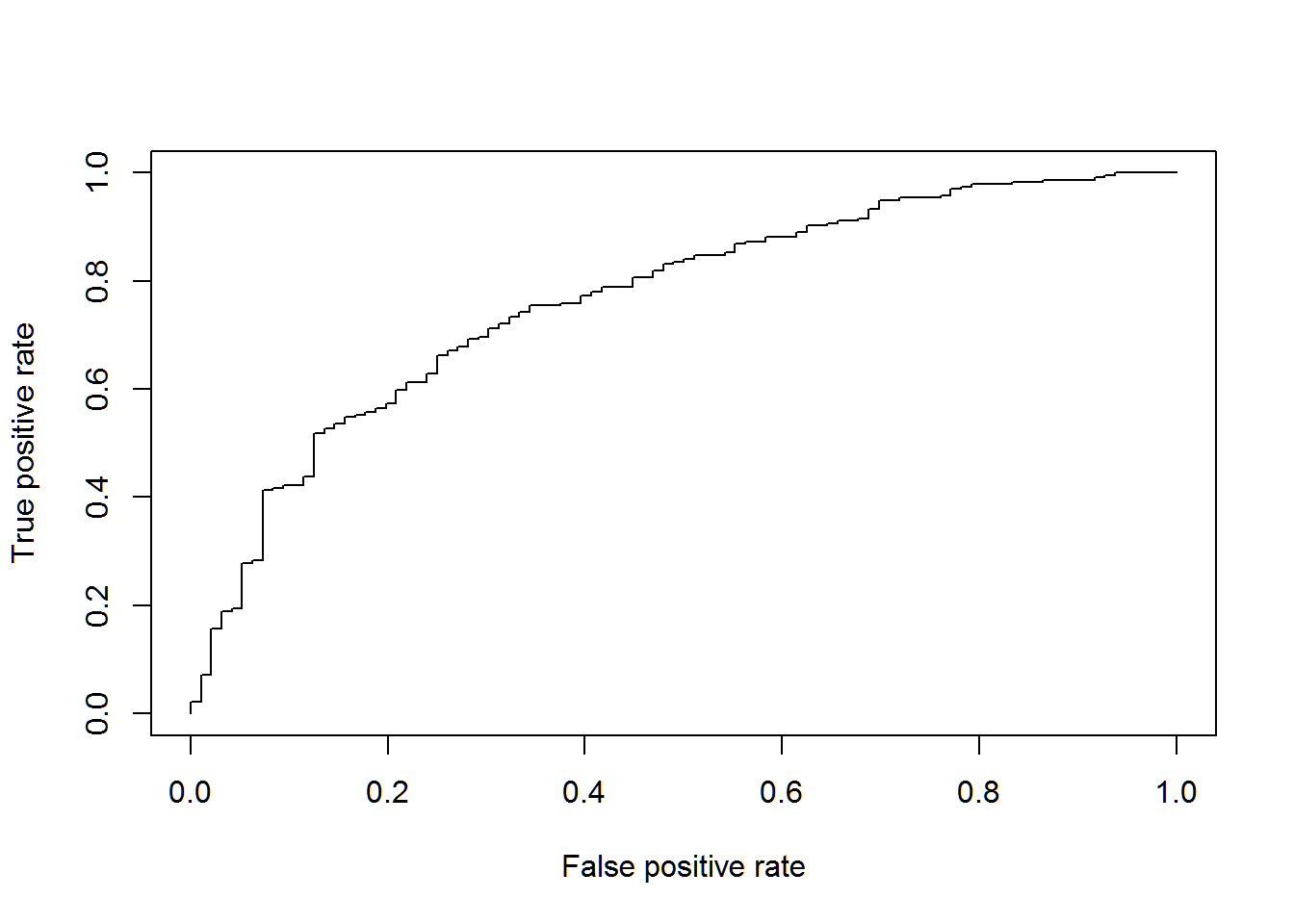AUCLog2 <- performance(pred2, measure = 'auc')@y.values[] AUCLog2 ##  0.7648998 We don’t get much improvement by including all the variables. A good rule to follow is keep the model as simple as possible. Adding more variables results in little improvement, so stick with the simpler model. #### Method Three: Regression Tree Next, let’s try analyzing the data using a regression tree approach. Much of our code is similar to what was used in the logistic models above but we need to do some tweaking, which you will recognize. Notice again we are looking at all the variables in our model to find their impact on our variable of interest, Creditability. It’s an awkward term which I think is probably a bad translation of the German word for Credit Worthiness. library(rpart) set.seed(1) TreeModel <- rpart(Creditability ~ ., data = credit[i_calibration1, ]) library(rpart.plot) prp(TreeModel, type = 2, extra = 1)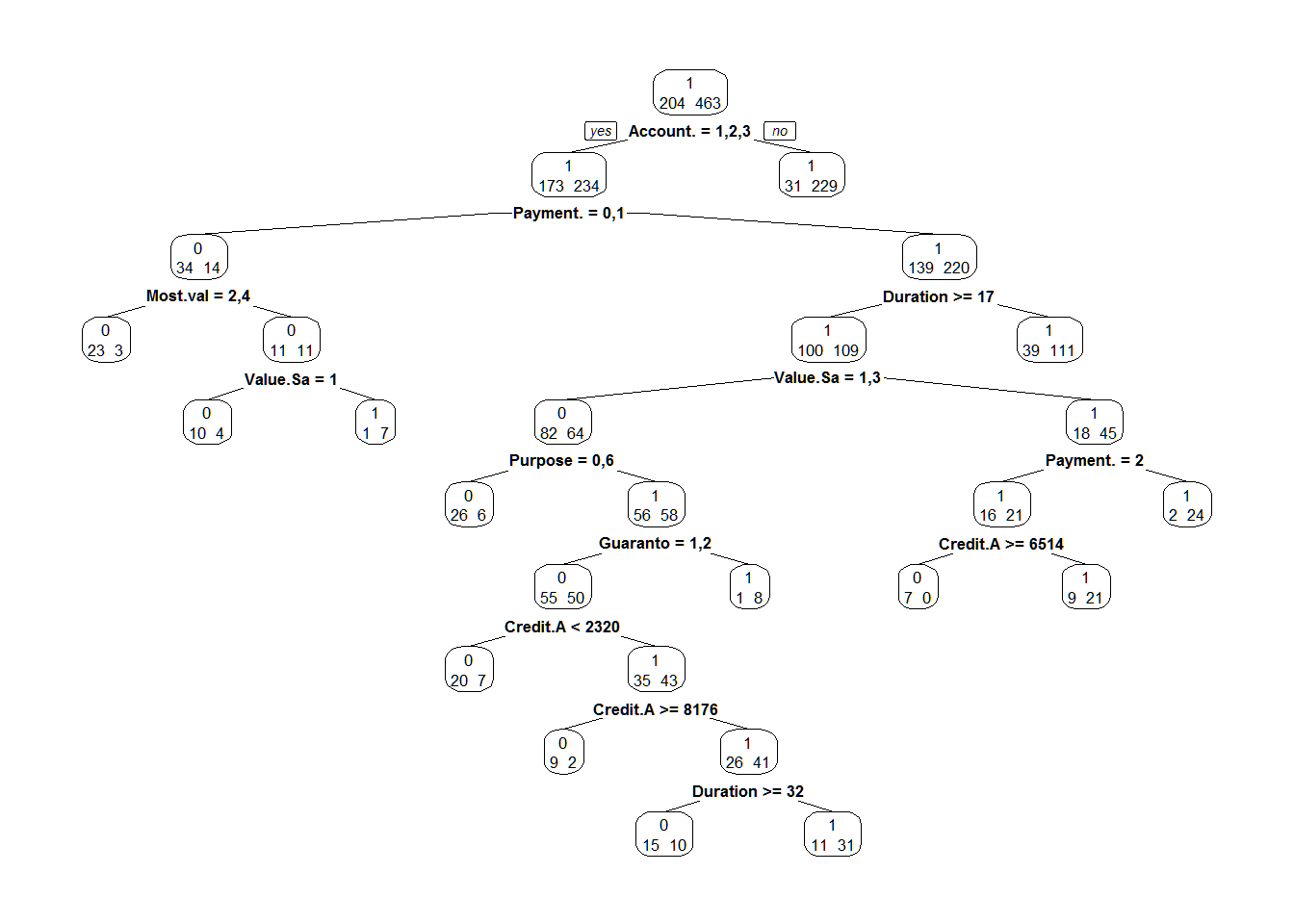fitTree <- predict(TreeModel, newdata = credit[i_test1, ], type = 'prob')[, 2] pred3 <- prediction(fitTree, credit$Creditability[i_test1])
perf3 <- performance(pred3, 'tpr', 'fpr')
plot(perf3)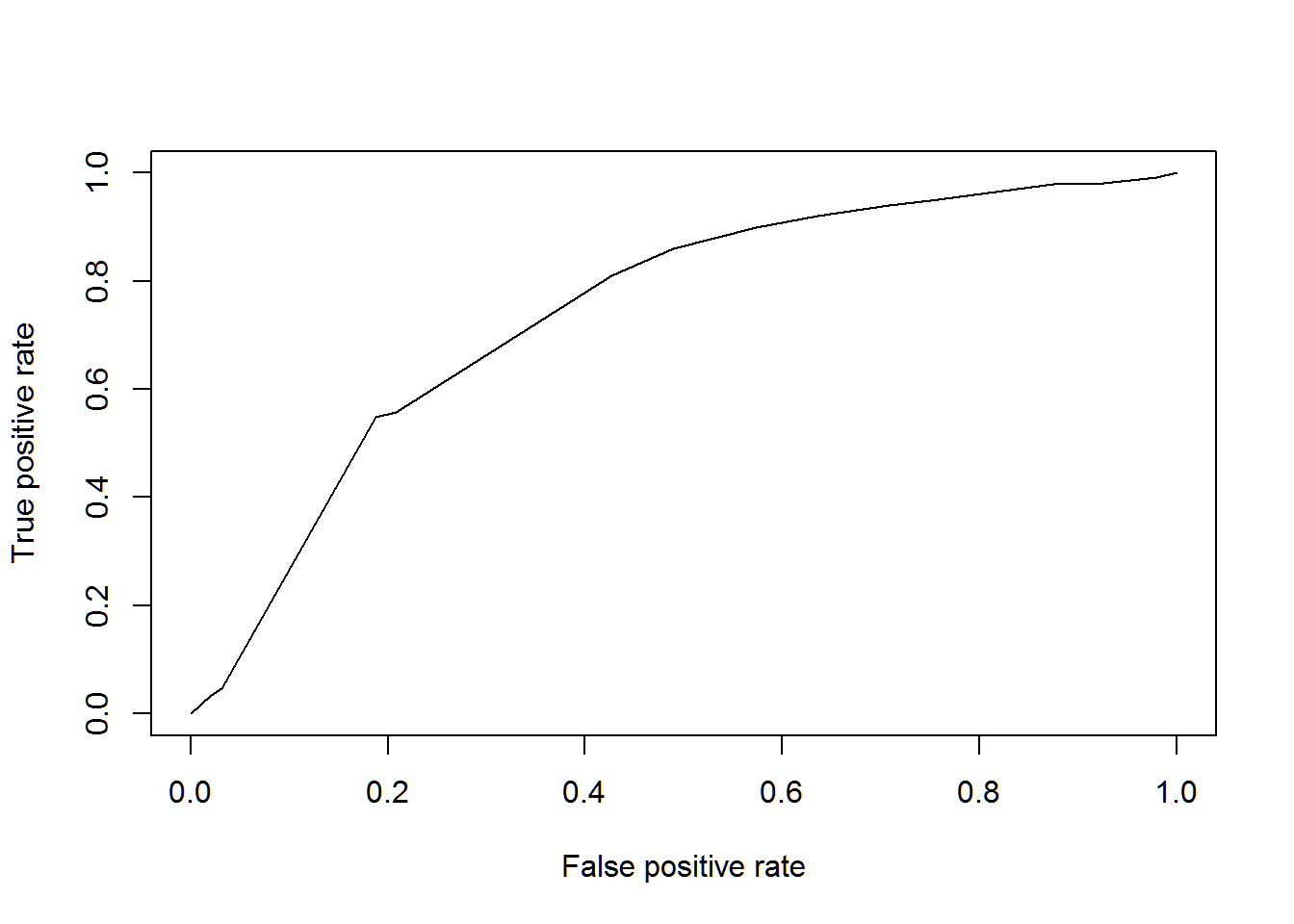AUCTree <- performance(pred3, measure = 'auc')@y.values[]
AUCTree
##  0.7414953

These aren’t satisfactory results, given all the complexity of our tree model, so again we have to wonder if we aren’t better off using the simpler Logistic Regression model from the first example.

#### Method Four: Random Forest

Instead of building one decision tree, we can use the random forest method to create a metaphorical “forest” of decision trees. In this method, the end result is the mode of the classes (if we are working on a classification model) or the mean of the predictions (if we are working with regressions).

The idea behind the random forest is that decision trees are prone to overfitting, so finding the “average” tree in the forest can help avoid this problem.

You can imagine this is more computationally demanding than creating a single decision tree, but R handles the effort pretty well.

library(randomForest)
set.seed(1)
RF <- randomForest(Creditability ~ ., data = credit[i_calibration1, ])
fitForest1 <- predict(RF, newdata = credit[i_test1, ], type = 'prob')[, 2]
pred4 <- prediction(fitForest1, credit$Creditability[i_test1]) perf4 <- performance(pred4, 'tpr', 'fpr') plot(perf4)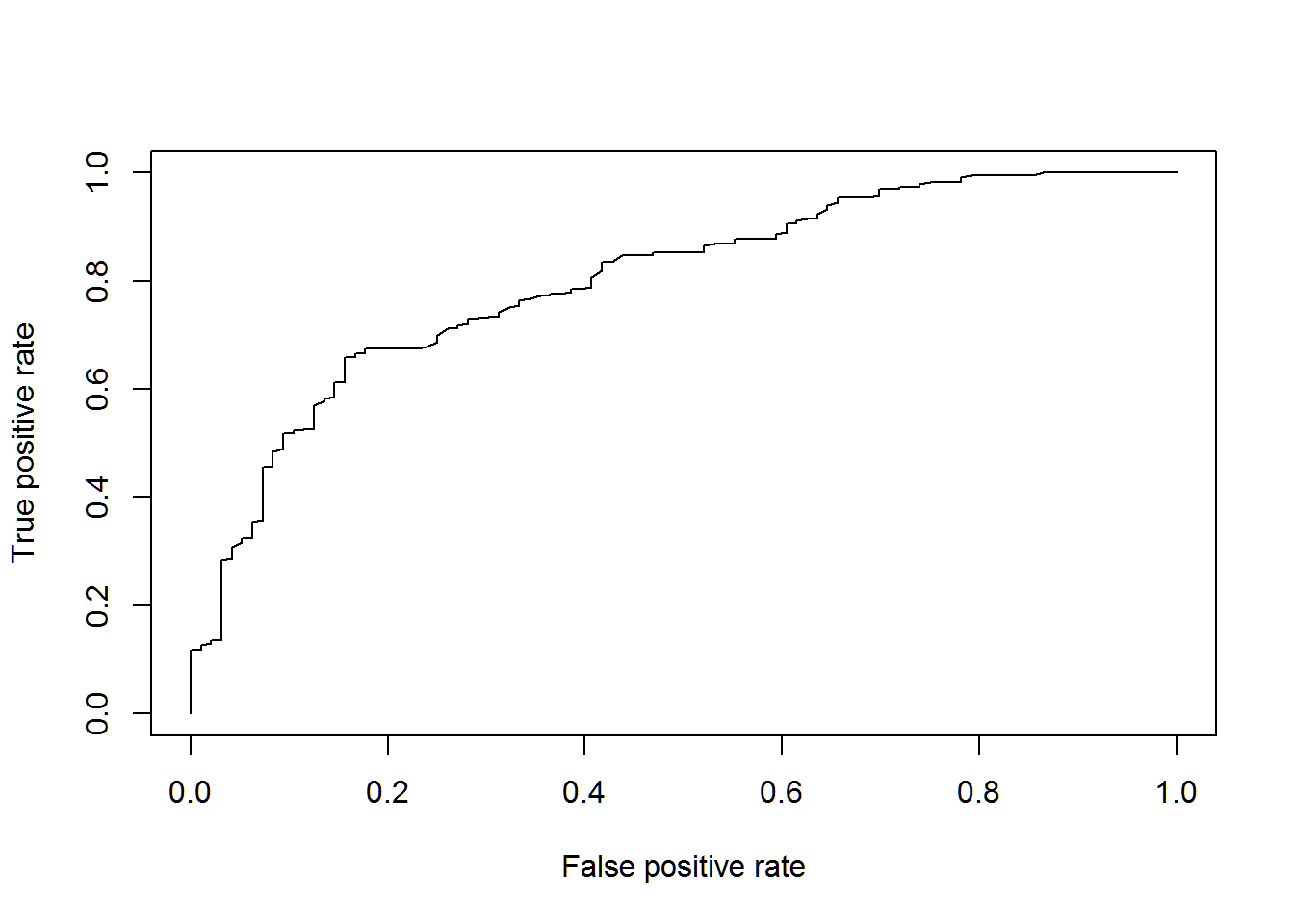AUCRF <- performance(pred4, measure = 'auc')@y.values[] AUCRF ##  0.7998637 With the extra effort, we get a somewhat improved result. The random forest model is the best performing of the four we have tried. But it’s a judgement call if the results warrant the extra work. #### Method Five: Comparing Random Forests with Logistic Models Alright, we’ve looked at various results using two basic methods of analysis – logistic regressions and decision trees. We have seen only single results expressed as AUC. The random forest approach requires we create a forest of decision trees and take the mode or average. Why not make use of all that data? What would they look like? The following code creates a chart depicting hundreds of combinations of AUC scores for each tree in our random forest and for logistic models. First we need a function to carry out the analysis. AUC <- function(i){ set.seed(i) i_test2 <- sample(1:nrow(credit), size = 333) i_calibration2 <- (1:nrow(credit))[-i_test2] LogisticModel.3 <- glm(Creditability ~ ., family = binomial, data = credit[i_calibration2, ]) summary(LogisticModel.3) fitLog3 <- predict(LogisticModel.3, type = 'response', newdata = credit[i_test2, ]) library(ROCR) pred5 <- prediction(fitLog3, credit$Creditability[i_test2])
AUCLog3 <- performance(pred5, measure = 'auc')@y.values[]
RF <- randomForest(Creditability ~ ., data = credit[i_calibration2, ])
fitForest2 <- predict(RF, newdata = credit[i_test2, ], type = 'prob')[, 2]
pred6 <- prediction(fitForest2, credit$Creditability[i_test2]) AUCRF <- performance(pred6, measure = 'auc')@y.values[] return(c(AUCLog3, AUCRF)) } OK, we have the function. Now we have to put it to work. This part of the code takes a while to run because we are tabulating and recording hundreds of individual results. You can adjust the number of results in the model by changing the count in the VAUC object. Here, we have chosen to calculate 200 x-y pairs, or 400 individual results. VAUC <- Vectorize(AUC)(1:400) plot(t(VAUC), xlab = 'Logistic', ylab = 'Random Forest')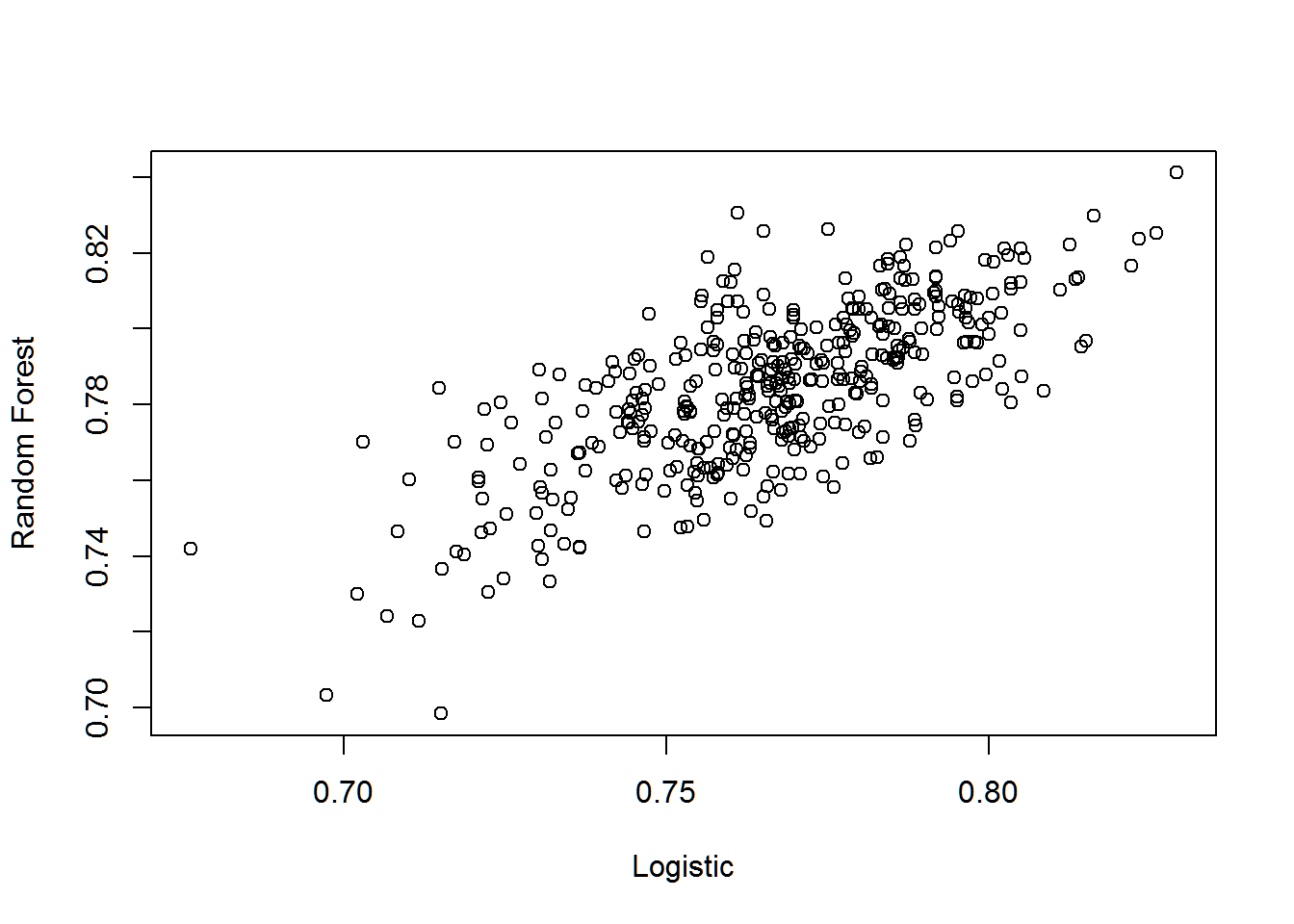You can see that the results we have from the first four models are right in the middle of the distribution. This confirms for us the models are all pretty comparable. The best we can hope for is an AUC of 0.84 and most give us results similar to what we’ve calculated already. But let’s try to visualize this a bit better. A couple R packages can be used to improve the graphic depiction of the results. This will show us exactly where the most likely set of results reside. First, we need to convert our VAUC object into a data frame. AA <- as.data.frame(t(VAUC)) We are going to use the ggplot2 and hdrcde libraries to create a couple new graphs. The first is a density contour plot. library(ggplot2) data(AA, package = 'MASS') ggplot(AA, aes(x = V1, y = V2)) + geom_point() + geom_density2d() + xlab('Logistic') + ylab('Random Forest')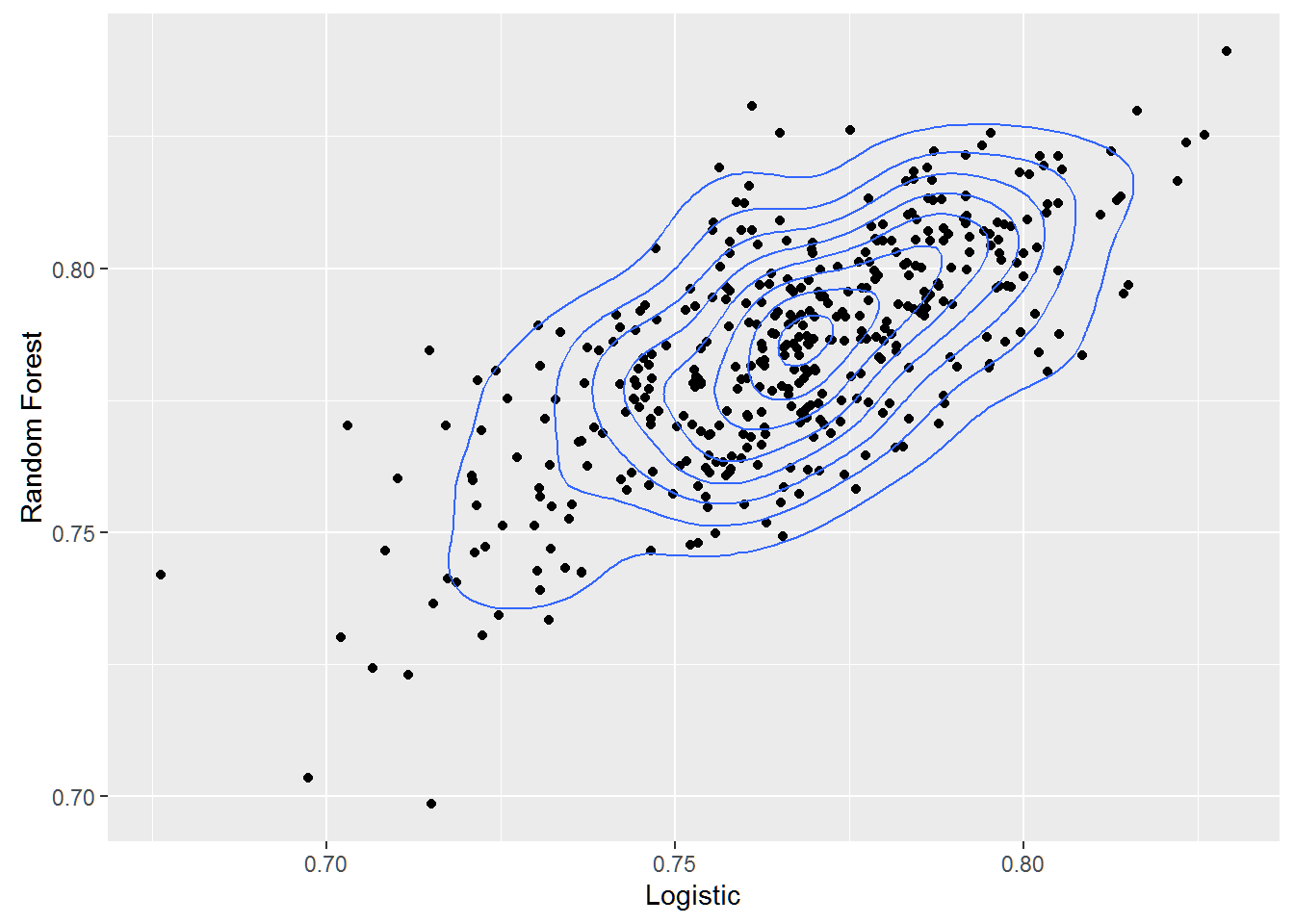The second is a high density contour plot that gives us the probability regions for the data. library(hdrcde) par(mar = c(3.1, 4.1, 1.1, 2.1)) with(AA, hdr.boxplot.2d(V1, V2, show.points = TRUE, prob = c(.01, .05, .5, .75), xlab = 'Logistic', ylab = 'Random Forest'))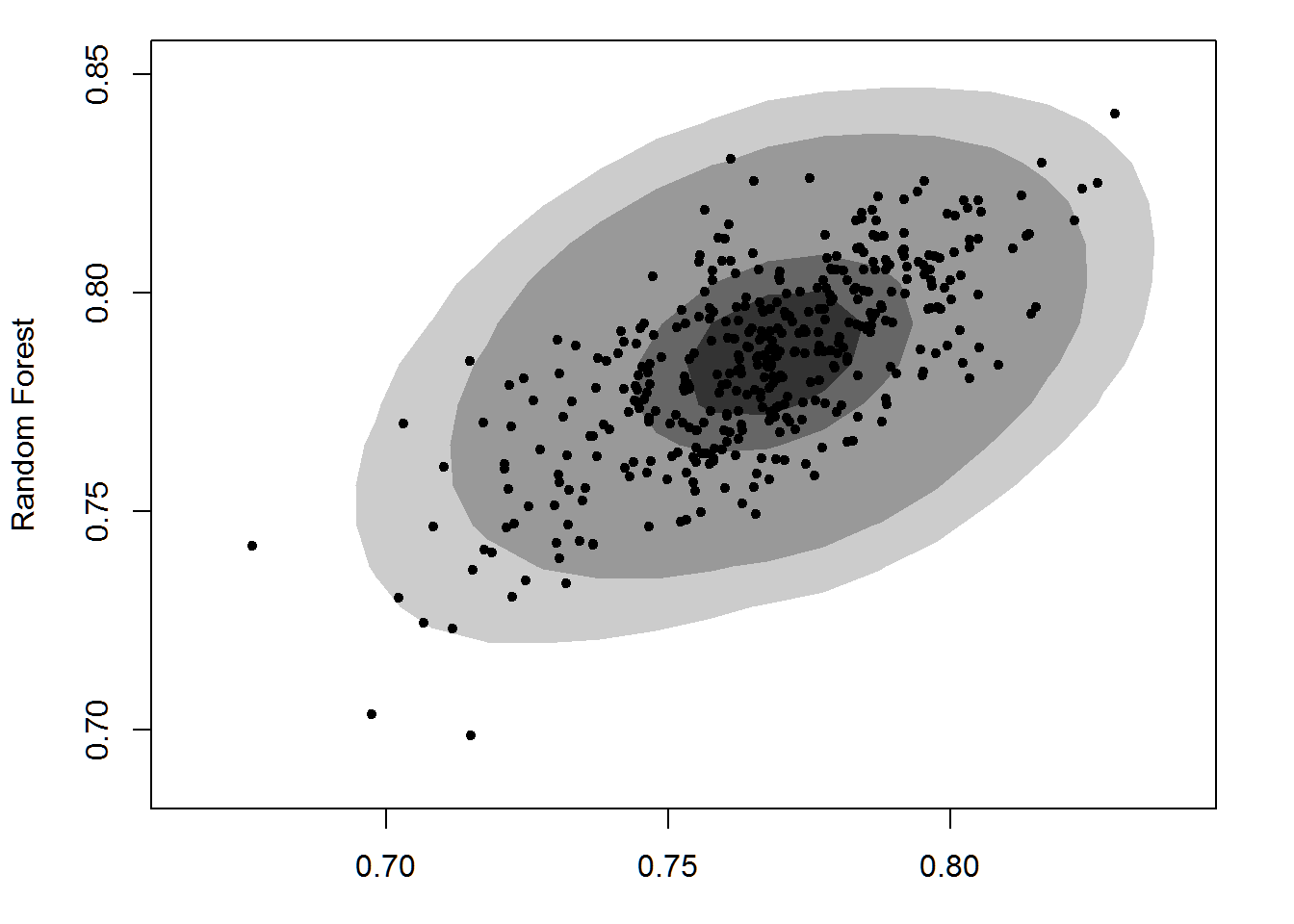Any way we depict our results, we have to use the data to make a sound lending decision. Is there a problem here? These may be the best scores we can come up with using these models, but are the results acceptable for determining the credit-worthiness of a loan applicant? That depends on the credit standards being used by the lending institution. At best, it looks like our models give us an 82% chance of lending to good credit risks. For every$1 million in loans, at best we might expect to be repaid $820,000. On average, we would expect to recover around$780,000 in principal. In other words, according to our analysis, there is between a 75% and 80% chance we will recapture our \$1 million loan, depending on the modeling method we use.

As we add loan applicants to our data bases, we would want them to cluster in the darkest area of the high density plot if we are going to consider them good credit risks.

Unless we charge a lot of interest to cover our losses, we might need better models.

The code in this example was adapted from Arthur Charpentier at the Freakonometrics blog in the post, Classification on the German Credit Database. The code is available at GitHub.